Courses

# Solutions of Reflection of Light (Page No- 179) - Physics By Lakhmir Singh, Class 10 Class 10 Notes | EduRev

## Class 10 Physics Solutions By Lakhmir Singh & Manjit Kaur

Created by: Ajay Batra

## Class 10 : Solutions of Reflection of Light (Page No- 179) - Physics By Lakhmir Singh, Class 10 Class 10 Notes | EduRev

The document Solutions of Reflection of Light (Page No- 179) - Physics By Lakhmir Singh, Class 10 Class 10 Notes | EduRev is a part of the Class 10 Course Class 10 Physics Solutions By Lakhmir Singh & Manjit Kaur.
All you need of Class 10 at this link: Class 10

Lakhmir Singh Physics Class 10 Solutions Page No:179

Question 5: Fill in the following blanks with suitable words :
Parallel rays of light are reflected by a concave mirror to a point called the………..
The focal length of a concave mirror is the distance from the………. to the mirror.
A concave mirror…….. rays of light whereas a convex mirror……… rays of light.
For a convex mirror, parallel rays of light appear to diverge from a point called the…………

Solution : (a) principal focus.
(b) principle focus.
(c) converges; diverges.
(d) principle focus.

Question 6: What is a spherical mirror ? Distinguish between a concave mirror and a convex mirror.

Solution : A spherical mirror is that mirror whose reflecting surface is the part of a hollow sphere of glass. The spherical mirrors are of two types: concave mirrors and convex mirrors.
Difference between concave mirror and convex mirror:
A concave mirror is that spherical mirror in which the reflection of light takes place at concave surface (or bent-in surface), whereas a convex mirror is that spherical miror in which the reflection of light takes place at the convex surface (or bulging out surface).
Concave mirror converges the parallel rays of light that fall on it, whereas convex mirror diverges the parallel rays of light that fall on it.

Question 7: Name the two types of spherical mirrors. What type of mirror is represented by the :
(a) back side of a shining steel spoon ?
(b) front side of a shining steel spoon ?

Solution : Two types of spherical mirrors are:
(i) Concave mirror
(ii) Convex mirror
Type of mirror represented by the:
(a) back side of a shining steel spoon – convex mirror
(b) front side of a shining steel spoon – concave mirror

Question 8: What is the relation between the focal length and radius of curvature of a spherical mirror (concave mirror or convex mirror) ? Calculate the focal length of a spherical mirror whose radius of curvature is 25 cm.

Solution : For a spherical mirror the principal focus (F) lies exactly mid-way between the pole (P) and centre of curvature (C). So, the focal length (f) of a spherical mirror is equal to half of its radius of curvature (R).
f = R/2
R = 25cm (Given)
f = ?
We know that
f = R/2
= 25/2
f = 12.5cm

Question 9: Explain with a suitable diagram, how a concave mirror converges a parallel beam of light rays. Mark clearly the pole, focus and centre of curvature of concave mirror in this diagram.

Solution :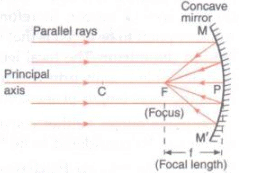All the light rays which are parallel to the principal axis of a concave mirror, converge at the the principal focus (F) after reflection from the mirror. Since a concave mirror converges a parallel beam of light rays, it is also called a converging mirror.

Question 10: Describe with a suitable diagram, how a convex mirror diverges a parallel beam of light rays. Mark clearly the pole, focus and centre of curvature of convex mirror in this diagram.

Solution :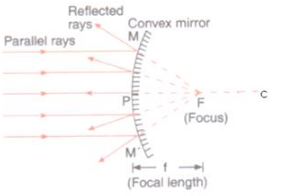All the light rays which are parallel to the principal axis of a convex mirror, appear to diverge from the principal focus (F) after reflection from the mirror. Since a convex mirror diverges a parallel beam of light rays, it is also called a diverging mirror.

Question 11: Define (a) centre of curvature (b) radius of curvature (c) pole (d) principal axis, and (e) aperture, of a spherical mirror with the help of a labelled diagram.

Solution :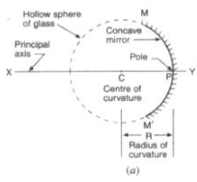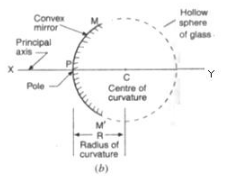(a) Centre of curvature: Centre of curvature of a spherical mirror is the centre of the hollow sphere of glass of which the mirror is a part.
(b) Radius of curvature: Radius of curvature of a spherical mirror is the radius of the hollow sphere of glass of which the mirror is a part.
(c) Pole: The centre of a spherical mirror is called its pole.
(d) Principal axis: The straight line passing through the centre of curvature and pole of a spherical mirror is called its principal axis.
(e) Aperture: The portion of a mirror from which the reflection of light actually takes place is called the aperture of the mirror.

Question 12: (a) Define (i) principal focus of a concave mirror, and (ii) focal length of a concave mirror.
Draw diagram to represent the action of a concave mirror on a beam of parallel light rays. Mark on this diagram principal axis, focus F, centre of curvature C, pole P and focal length ƒ, of the concave mirror.

Solution : (a) (i) Principal focus of a concave mirror: The principal focus of a concave mirror is a point on its principal axis to which all the light rays which are parallel and close to the axis, converge after reflection from the concave mirror.
(ii) Focal length of a concave mirror: The focal length of a concave mirror is the distance between its pole and the principal focus.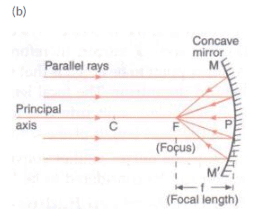Question 13: (a) What is meant by (i) principal focus of a convex mirror, and (ii) focal length of a convex mirror ?
(b) Draw diagram to show the action of convex mirror on a beam of parallel light rays. Mark on this diagram principal axis, focus F, centre of curvature C, pole P and focal length ƒ, of the convex mirror.

Solution : (a) (i) Principal focus of a convex mirror: The principal focus of a convex mirror is a point on its principal axis from which a beam of light rays, initially parallel to the axis, appears to diverge after being reflected from the convex mirror.
(ii) Focal length of a concave mirror: The focal length of a convex mirror is the distance from the pole (P) to its principal focus (F).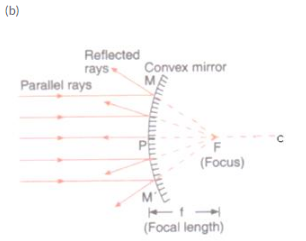Question 19: A communications satellite in orbit sends a parallel beam of signals down to earth. If these signals obey the same laws of reflection as light and are to be focussed onto a small receiving aerial, what should be the best shape of the metal ‘dish’ used to collect them ?

Solution : Concave metal dish: It will collect the parallel beam of satellite signals at its focus where receiving aerial is fixed.

Question 20: When a spherical mirror is held towards the sun and its sharp image is formed on a piece of carbon paper for some time, a hole is burnt in the carbon paper.
(a) What is the nature of spherical mirror ?
(b) Why is a hole burnt in the carbon paper ?
(c) At which point of the spherical mirror the carbon paper is placed ?
(d) What name is given to the distance between spherical mirror and carbon paper ?
(e) What is the advantage of using a carbon paper rather than a white paper ?

Solution : (a) Concave mirror
(b) A lot of sun’s heat rays are concentrated at the point of sun’s image which burn the hole in carbon paper.
(c) At the focus.
(d) Focal length.
(e) A black carbon paper absorbs more heat rays and hence burns a hole more easily (than a white paper).

Offer running on EduRev: Apply code STAYHOME200 to get INR 200 off on our premium plan EduRev Infinity!

94 docs

,

,

,

,

,

,

,

,

,

,

,

,

,

,

,

,

,

,

,

,

,

,

,

,

;